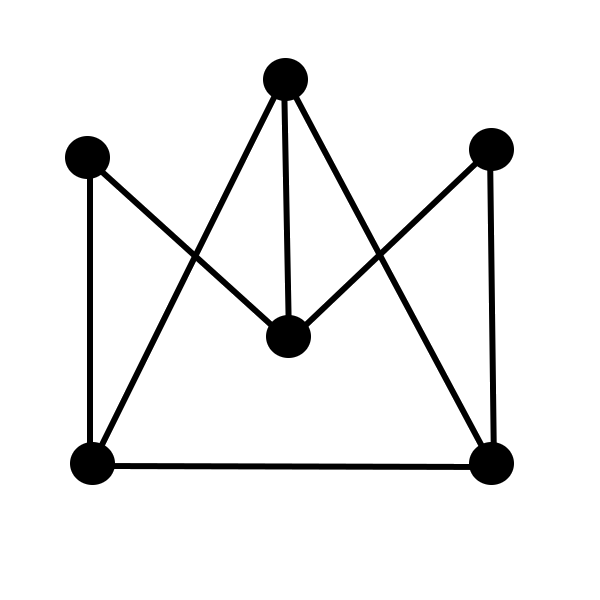MTH4140 Home

# MTH4140: Midterm 2 Practice 2

Problem 1

Provide an example of a graph whose chromatic number is 5

Problem 2

Construct an example of a simple graph $$G$$ that has $$6$$ vertices and $$9$$ edges. Determine $$\omega(G)$$ of the graph $$G$$ that you constructed.

Problem 3

Determine the chromatic polynomial of the graph belowProblem 4

Assume that the chromatic number of the simple graph $$H$$ is equal to $$k$$ and that $$H$$ is a $$k$$-critical graph. Prove that the following inequality holds $\delta(H)\geq k-1,$ where $$\delta(H)$$ is the minimum of the degrees of vertices in $$H$$.

Problem 5

Assume that a simple graph $$G$$ has exactly $$52$$ vertices and the set of vertices has three disjoint subsets $$T_1$$, $$T_2$$, and $$T_3$$ that satisfy the following two properties:

• (i) Each of the subsets $$T_1$$, $$T_2$$, and $$T_3$$ has $$5$$ vertices;

• (ii) For each $$i\in\{1,2,3\}$$ no two vertices of $$T_i$$ are adjacent.

Prove that $$\chi(G)\leq 40$$.